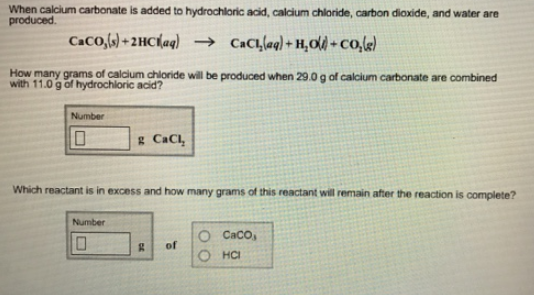# Problem: When calcium carbonate is added to hydrochloric acid, calcium chloride, carbon dioxide, and water are produced.CaCO3 (s) + 2HCl (aq) → CaCI2 (aq) + H2O (l) + CO2 (g) How many grams of calcium chloride will be produced when 29.0 g of calcium carbonate are combined with 11.0 g of hydrochloric acid? Which reactant is in excess and how many grams of this reactant will remain after the reaction is complete?

###### FREE Expert Solution
81% (125 ratings)###### Problem Details

When calcium carbonate is added to hydrochloric acid, calcium chloride, carbon dioxide, and water are produced.

CaCO3 (s) + 2HCl (aq) → CaCI2 (aq) + H2O (l) + CO2 (g)

How many grams of calcium chloride will be produced when 29.0 g of calcium carbonate are combined with 11.0 g of hydrochloric acid?

Which reactant is in excess and how many grams of this reactant will remain after the reaction is complete?